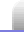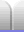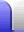# Triangles

### Types of Triangles

The angles inside a triangle are supplementary (they total 180°). We normally just say the angles of a triangle add up to 180° (or "sum to 180°").

 A right triangle has one angle which is 90°. The side opposite the right angle is the longest side of the triangle and is called the hypotenuse. See Pythagoras' Theorem for more about right triangles. In a right angle triangle the two non-right angles are complementary (they total 90°).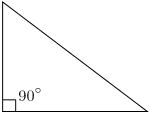An acute triangle has all its angles less than 90°.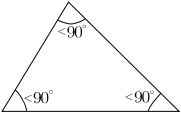An equilateral triangle is another sort of acute triangle, in which all three angle and all three sides are equal.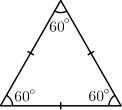An obtuse triangle has one angle which is more than 90°.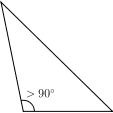An isosceles triangle has two angles and two sides equal and can be an acute triangle or an obtuse triangle. (The two base angles will always be acute because they must sum to <180°, meaning they must each be less than 90°.)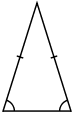What will a right isosceles triangle look like? A scalene triangle has no sides the same length (which means the angles will also all be different). Scalene triangles can be right or acute or obtuse.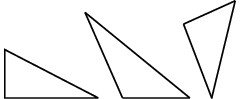### Area of a Triangle

• The simplest way of calculating the area of a triangle is the formula:

A = ½bh

Where b is the base length and h is the perpendicular height.

• If you know the length of two sides and the angle between them there is a more generalised formula:

A = ½absinC

Where a and b are the two known sides and C is the angle between them (and opposite the third side, c). The reason it works is that if b is the base, the perpendicular height h = a·sinC.

The formula also works for the area of a quadrilateral where a and b are the diagonals and C is the angle between them.

• If you know the length of the three sides there is Heron's formula, named after Hero of Alexandria. It's not part of any secondary school syllabus, but it's quite handy because it does not depend on knowing any angles: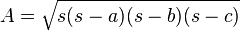Where s is half the perimeter: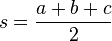Heronian triangles have their sides and area which are all whole numbers. All Pythagorean triangles are also Heronian triangles.Home Astronomy Chemistry Electronics & Computers Mathematics Physics Field Trips Turn on javascript for email link